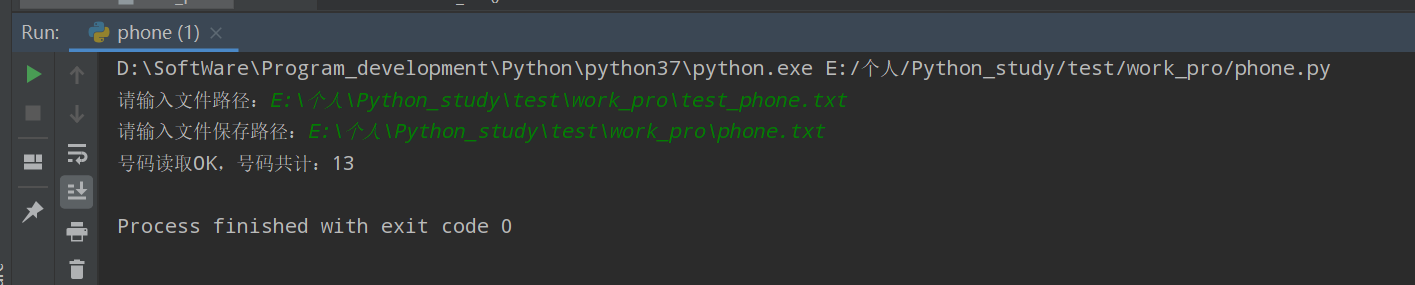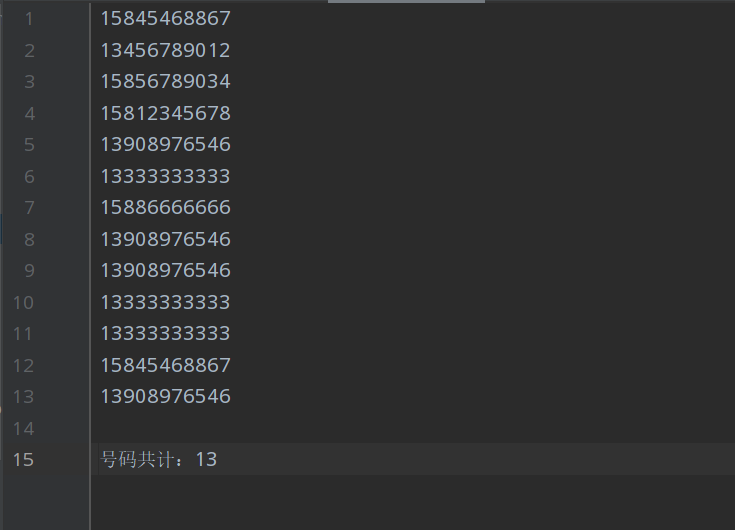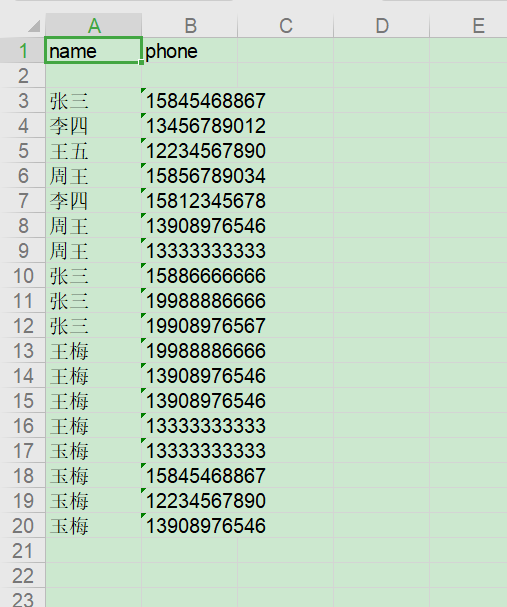## Python 打怪兽之计算中文字数

 `12345678910111213141516` ``````#coding:utf-8 import re #读取目标文本文件 def get_str(path): f = open(path) data = f.read() f.close() return data # 输入目标路径 path=input("请输入文件路径：") word=re.findall('([\u4e00-\u9fa5])',get_str(path)) # 计算出特殊字符外的字数 print("中文字符,除特殊字符外共：",len(word)) ``````## Python 打怪兽之提取文本中手机号

### 将提取的手机号存入txt

 `123456789101112131415161718192021222324252627282930` ``````import re #读取目标文本文件 def get_str(path): f = open(path,encoding="utf-8") data = f.read() f.close() return data # 正则获取文本号码 def get_phone_number(str): res = re.findall(r'(13\d{9}|14[5|7]\d{8}|15\d{9}|166{\d{8}|17[3|6|7]{\d{8}|18\d{9})', str) return res #保存得到号码 def save_res(res,save_path): save_file = open(save_path, 'w') for phone in res: save_file.write(phone) save_file.write('\n') save_file.write('\n号码共计：'+str(len(res))) save_file.close() print('号码读取OK，号码共计：'+str(len(res))) if __name__ == '__main__': path=input("请输入文件路径：") save_path=input("请输入文件保存路径：") #read_str=get_str(path) res=get_phone_number(get_str(path)) save_res(res,save_path) ``````### 将提取的手机号存入 Excel

 `123456789101112131415161718192021222324252627282930313233343536373839404142434445464748495051` ``````#coding:utf-8 import xlwt #读取目标文本文件 def get_str(path): f = open(path, encoding="utf-8") data = f.read() f.close() return data def save_excel(save_path,sheetname,column_name_list,read_list): workbook = xlwt.Workbook() sheet1 = workbook.add_sheet(sheetname=sheetname) for i in range(0,len(column_name_list)): sheet1.write(0,i,column_name_list[i]) i = 1 for v in read_list: kval = v.split('：') for j in range(0, len(kval)): sheet1.write(i + 1, j, kval[j]) print(kval[j]) i = i + 1 #保存为Excel文件 def save_excel(save_path,sheetname,column_name_list,read_list): workbook = xlwt.Workbook() sheet1 = workbook.add_sheet(sheetname=sheetname) for i in range(0,len(column_name_list)): sheet1.write(0,i,column_name_list[i]) i=1 for v in read_list: kval=v.split('：') for j in range(0,len(kval)): sheet1.write(i+1,j,kval[j]) i=i+1 workbook.save(save_path) print('信息保存 OK，记录条数共计：'+str(len(read_list))) if __name__ == '__main__': path = input("请输入文件路径：") save_path = input("请输入文件保存路径：") sheet_name = input("请输入sheetname：") column_name = input("请输入列名，并且使用英文逗号隔开：") column_name_list = column_name.split(',') read_str = get_str(path) read_list = read_str.split('\n') save_excel(save_path, sheet_name, column_name_list, read_list) ``````### 总结